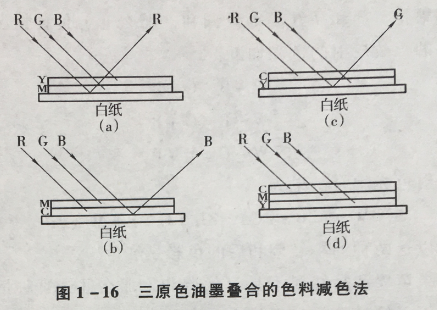### | 一站式印刷包装解决方案专家

400-021-1886 186 1663 4825

7X24小时服务热线

## 全部印刷品

 首页 >> 印刷专题 >>包装印刷 >> 什么是包装袋印刷网点叠合呈色？

# 什么是包装袋印刷网点叠合呈色？Y=W-B

M=W-G

Y+M=W-B-G=R

M=W-G

C=W-R

M+C=W-G-R=B

C=W-R

Y=W-B

C+Y=W-R-B=G

Y=W-B

M=W-G

C=W-R

Y+M+C=W-B-G-R=W-（B+G+R）=00
×

### 购物车